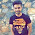### Algorithm Puzzle: Triplets in Array

Source: Asked to me by Anuj Jain (EE IITB 2010 Graduate, MFE Student at Baruch College NY)

Problem:
Given an array of n integers, find an algorithm to find triplets in the array such that sum of the three numbers is zero.

What is the order of your algorithm? Make sure its quadratic in size of array. :-)

1.Have a solution in quadratic time and linear extra space:

Step 1: Sort the array - O(n^2) time

Step 2: Find the largest negative number in the array (there has to be one for such a triplet to exist) - O(log n) time

Step 3: Iterate over the negative numbers like this:

For every negative number k, look for existence of two numbers whose difference is -k.

To do this, subtract n from every integer in array and copy to second array. Now do a pass over both arrays similar to a pass done while merging two sorted arrays.

In this way we can get two numbers whose difference is -k in 0(n) time and O(n) space. And we do this for every negative number. So total order is O(n^2)...

Hence total time is O(n^2) and space is O(n).

Btw we can easily forgo O(n) space as well, just by remembering to add k to numbers in second array while comparing...

2.sort the array; O(nlogn)

now for i = 1,2..N

find a(j) and a(k) (j,k >i) such that a(j)+a(k)=-a(i)

this can be done in O(n) time as follows

let y = -a(i)
j = (i+1)
k = (n)

if y = a(j)+a(k) return (j,k);
if y> a(j)+a(k) j=j+1;
if y< a(j)+a(k) k = k-1;

3.Create 2 arrays from the input Array

A with all elements >= 0
B with all elements < 0 [n]

Sort both the arrays (merge-sort) [WorstCase: 2n.log(n)]
//A is sorted in decending order, B in Asscending

i=0, j=0

while(i < A.length && j < B.length)
{

if (A[i] > -B[j])
{
Let X = -A[i] -B[j]
Search for X in Array B. (binary search, B is sorted) [log(n)]

if (X is found at index k)
print(A[i],B[j],B[k]);

i++;
}
else
{
Let X = -A[i] +B[j]
Search for X in Array A. (binary search, A is sorted) [log(n)]

if (X is found at index k)
print(A[i],B[j],A[k]);

j++;
}

}

//the total complexity of above while loop [n.log(n)]

============================================

time complexity slightly greater than 3n.log(n) + n

4.Sort the elements,in O(nlogn) time, in increasing order.
Let sum be S=0.
For each element in the array A_i (for i from 1 to N)
Compute S' = -A_i.
left = i+1
right = n
while(leftS') \\Means you need to reduce the number.
right--
else
left++
end while
end for

The while loop takes only O(n) time.
This can be seen by understanding that if a certain number can form a triplet, it will NOT be skipped.
and since each step, left and right comes closer by 1 the order is O(n)

5.setofTriplets = []
def findTriplets(array):
global setofTriplets
array.sort()
for i in range(len(array)):
for j in reversed(range(len(array))):
if array[i]<0 and array[j]>=0:
sum = array[i]+array[j]
needToFind = -1 * (array[i] + array[j])
if needToFind in array:
ans = [array[i], array[j], needToFind]
setofTriplets.append(ans)

findTriplets([-9, -4, -3, -1, 0, 1, 2, 6, 7, 8])
print setofTriplets